# Aquarium Control & Monitoring

Controlling lights and CO2 admission to a aquarium. Sensor data is stored so it can be used for statistics.

IntermediateShowcase (no instructions)7,145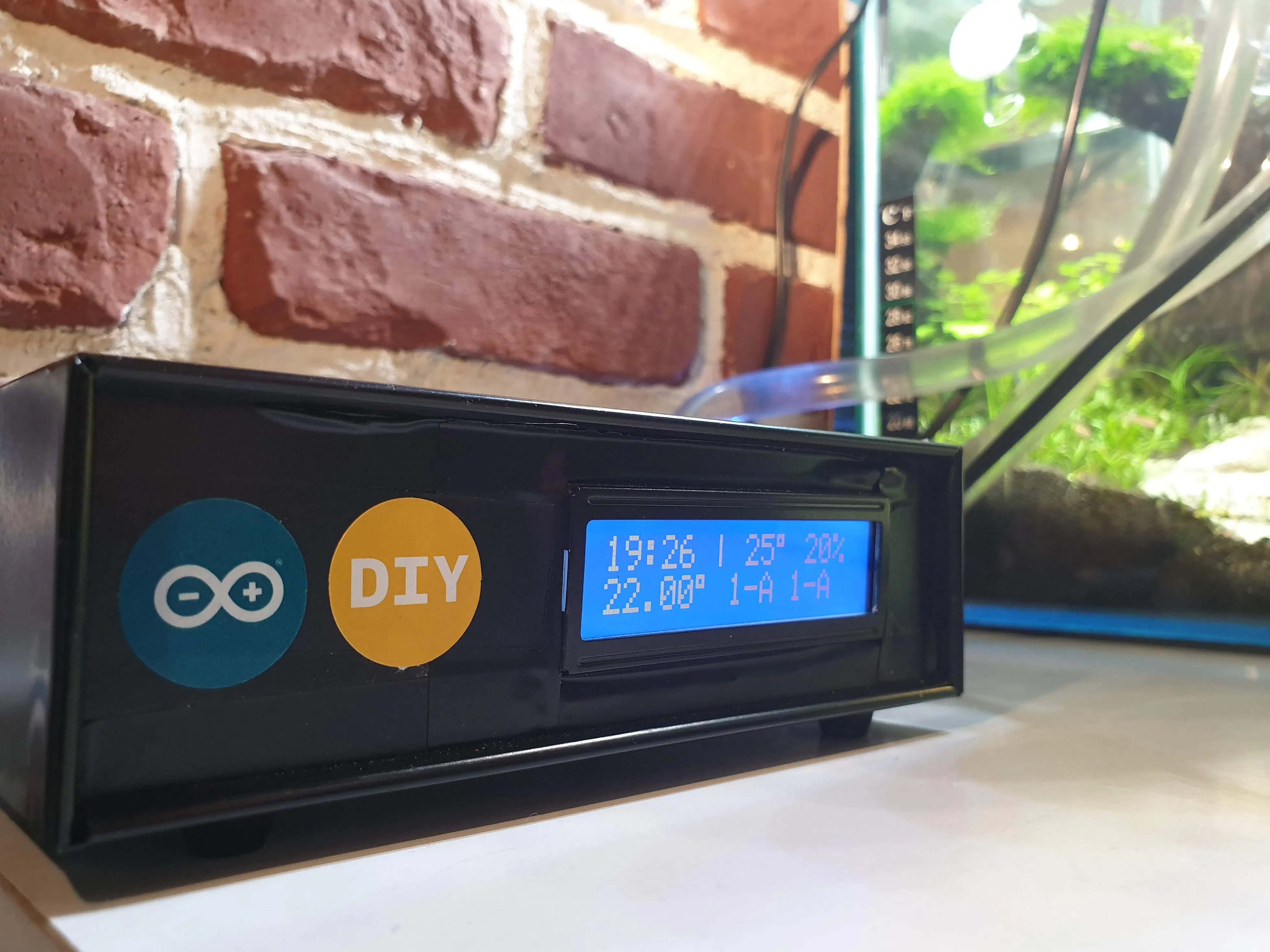## Things used in this project

### Hardware componentsArduino UNO & Genuino UNO
×1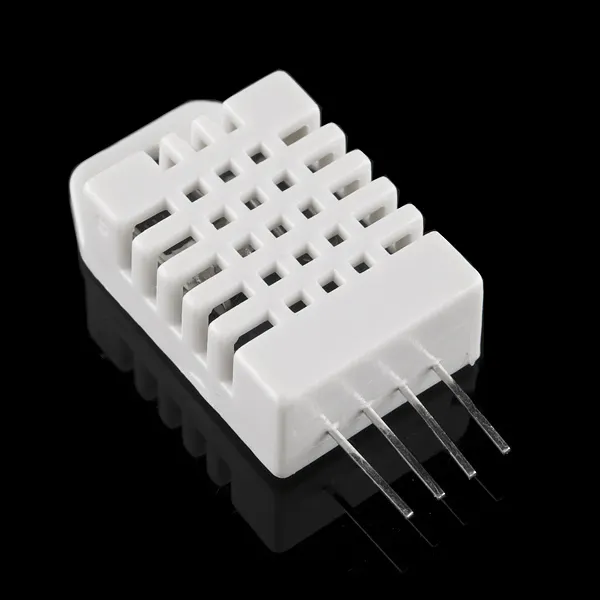DHT22 Temperature Sensor
×1
 Infrared Module (Generic)
×1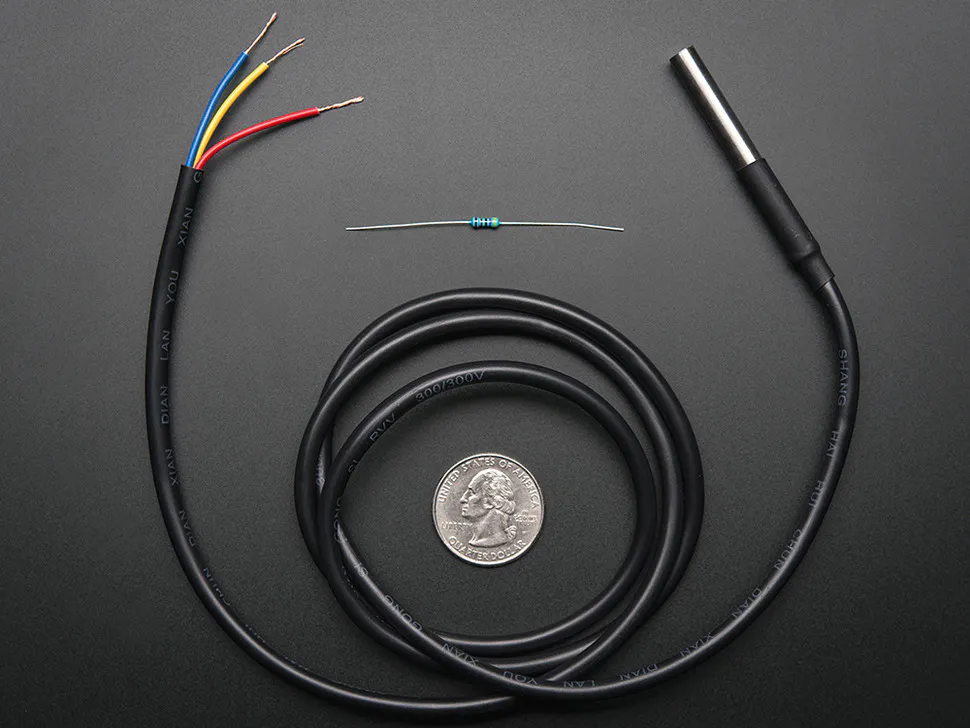Adafruit Waterproof DS18B20 Digital temperature sensor
×1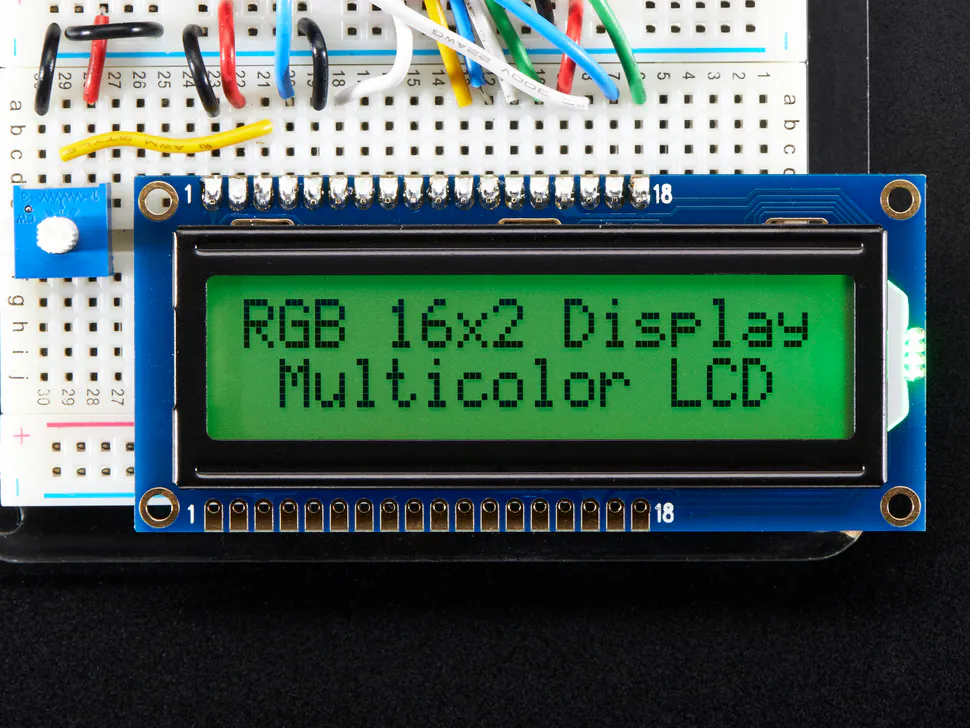Adafruit RGB Backlight LCD - 16x2
×1
 2-Channel SPDT Relay Carrier with 5VDC Relays
×2
 SparkFun RTC Module
×1Resistor 10k ohm
×1
 Resistor 4.7k Ohm
×1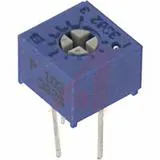Single Turn Potentiometer- 10k ohms
×1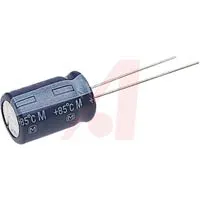Capacitor 10 µF
×1
 Metalic Case
×1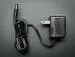9V 1A Switching Wall Power Supply
×1×1

### Hand tools and fabrication machinesSoldering iron (generic)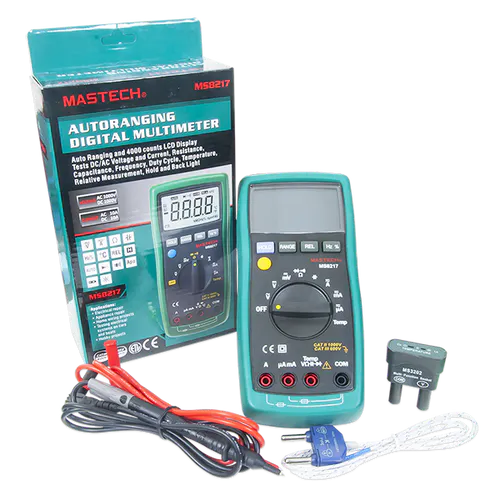Digilent Mastech MS8217 Autorange Digital MultimeterHot glue gun (generic)

## Schematics

### Fritzing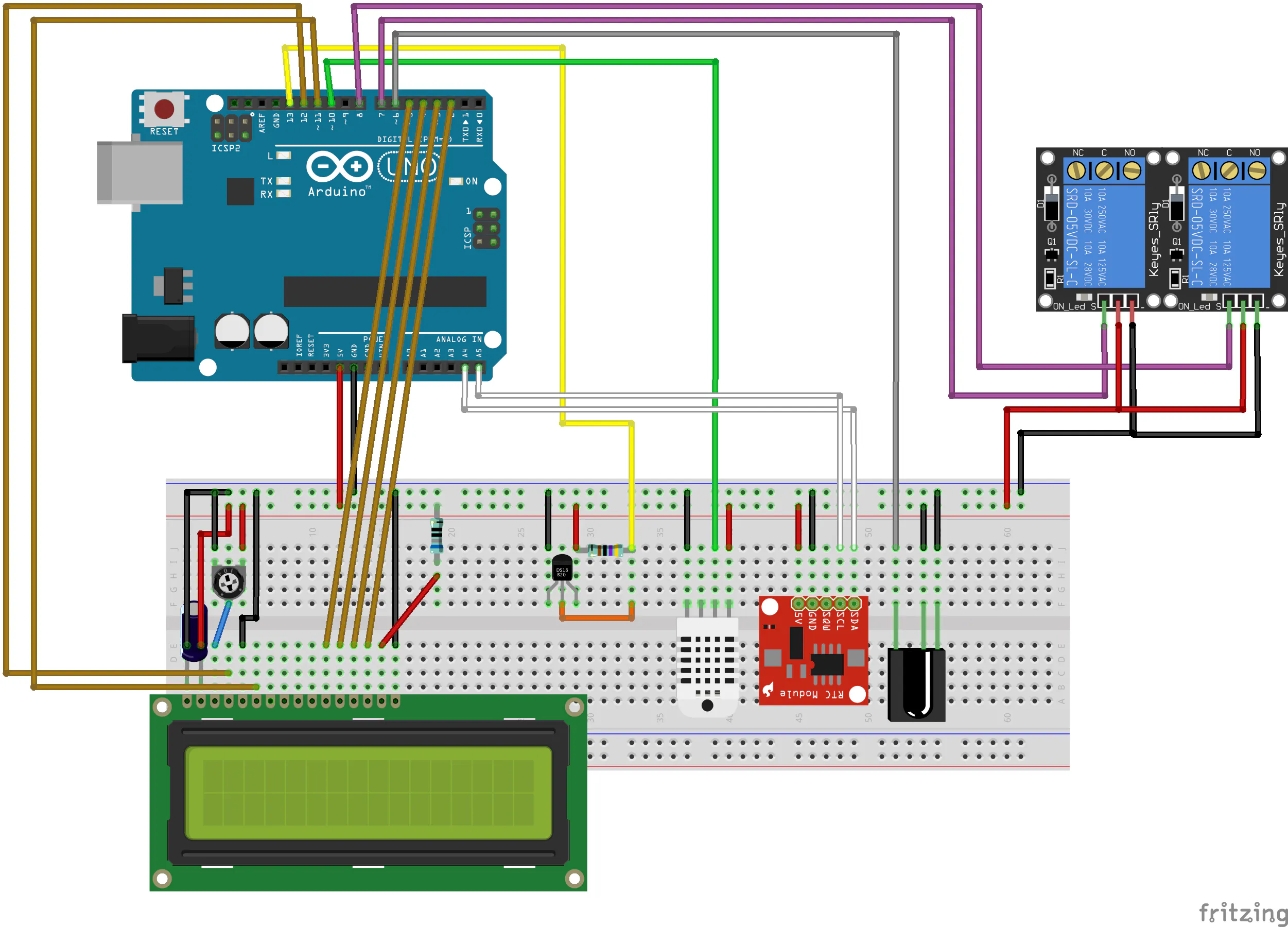## Code

### Sketch File

Arduino
```#include "RTClib.h"
#include <Time.h>
#include <TimeAlarms.h>
#include <DHT.h>
#include <OneWire.h>
#include <DallasTemperature.h>
#include <LiquidCrystal.h>
#include <EEPROM.h>
#include "IRremote.h"

// RTC
RTC_DS1307 rtc;

// DHT humidity senzor
#define DHTPIN 10     // what pin we're connected to
#define DHTTYPE DHT22   // DHT 22  (AM2302)
DHT dht(DHTPIN, DHTTYPE); //// Initialize DHT sensor for normal 16mhz Arduino

// Water proof senzor DS18B20
#define ONE_WIRE_BUS 13
OneWire oneWire(ONE_WIRE_BUS);
DallasTemperature sensors(&oneWire);

// LCD screen
LiquidCrystal lcd(12, 11, 5, 4, 3, 2);
byte degSymbol = {
B11100,
B10100,
B11100,
B00000,
B00000,
B00000,
B00000,
};
int lcdPage = 0;

// IR
int irPin = 6;
IRrecv irrecv(irPin);
decode_results irResults;
long irKey = 0;

char rx_byte = 0;
String key = "";
bool isKey = false;
String value = "";
bool isValue = false;

// Aquarium LED default values
int led1OnH = 8;
int led1OnM = 30;
int led1OffH = 12;
int led1OffM = 30;
int led2OnH = 18;
int led2OnM = 30;
int led2OffH = 20;
int led2OffM = 0;

// Aquarium CO2 default values
int co2OnH = 7;
int co2OnM = 0;
int co2OffH = 21;
int co2OffM = 0;

// Aquarium options
bool ledAuto = true;
bool co2Auto = true;
bool co2On = false;
bool ledOn = false;

// Aquarium status
float aquariumTemp;

// Ambient status
float ambientTemp, ambientHum;

// Status send to DB every 15 mins
int statusSendMinutes = 20;

// Alarms
AlarmID_t led1OnAlarm = 0;
AlarmID_t led1OffAlarm = 0;
AlarmID_t led2OnAlarm = 0;
AlarmID_t led2OffAlarm = 0;
AlarmID_t co2OnAlarm = 0;
AlarmID_t co2OffAlarm = 0;

// Relay pins
int relayLed = 7;
int relayCo2 = 8;

void setup() {
pinMode(relayLed, OUTPUT);
pinMode(relayCo2, OUTPUT);

// Use only without RTC
// setTime(10,19,0,16,2,19);

Serial.begin(9600);
dht.begin();
sensors.begin();

lcd.createChar(0, degSymbol);
lcd.begin(16, 2);

// Start Ir reciever

if (! rtc.begin()) {
Serial.println("Couldn't find RTC");
while (1);
}

if (! rtc.isrunning()) {
Serial.println("RTC is NOT running!");
}

// Sync time lib with RTC
setSyncProvider(getRTCTime);
setSyncInterval(60 * 60 * 4);

// should run only once at initial setup
// writeSettingsFromMemory();

updateLedState();
updateCo2State();
updateRelay();
sendStatus();

setAlarms();
Alarm.timerRepeat(60 * statusSendMinutes, sendStatus);
Alarm.timerRepeat(60, updateLcd);
}

void loop() {
while(Serial.available()){
// Read seril data and create key values pairs

if (rx_byte != '\n') {
if (rx_byte == '\$') {
isValue = false;
isKey = true;
continue;
}
if (rx_byte == '=') {
isValue = true;
isKey = false;
continue;
}
if (rx_byte == '&') {
setData();

value = "";
key = "";
isValue = false;
isKey = false;
continue;
}

if (!isKey) {
value += rx_byte;
} else {
key += rx_byte;
}
} else {
if (isValue) {
setData();

if (key != "" && key != "ledOn" && key != "co2On") {
updateLedState();
updateCo2State();
updateAlarms();
updateRelay();
}
}

value = "";
key = "";
isKey = false;
isValue = false;
sendStatus();
}
}

// IR remote loop
if (irrecv.decode(&irResults)) {
dumpIR(&irResults);

irrecv.resume(); // Receive the next value
//    Serial.println(irKey, HEX);
//    Serial.println();

if (irKey == 16720605) {
toggleLed();
} else if (irKey == 16712445) {
toggleCo2();
} else if (irKey == 16724175) {
loopLcdPage(-1);
} else if (irKey == 16743045) {
loopLcdPage(1);
}
}

Alarm.delay(1000);
}

void dumpIR(decode_results *irResults) {
int count = irResults->rawlen;
irKey = irResults->value;
}

void setAlarms() {
led1OnAlarm = Alarm.alarmRepeat(led1OnH, led1OnM, 20, LedOn);
led1OffAlarm = Alarm.alarmRepeat(led1OffH, led1OffM, 20, LedOff);

if (led2OnH != 0) {
led2OnAlarm = Alarm.alarmRepeat(led2OnH, led2OnM, 20, LedOn);
led2OffAlarm = Alarm.alarmRepeat(led2OffH, led2OffM, 40, LedOff);
}

co2OnAlarm = Alarm.alarmRepeat(co2OnH, co2OnM, 40, Co2On);
co2OnAlarm = Alarm.alarmRepeat(co2OffH, co2OffM, 40, Co2Off);
}

void updateAlarms() {
Alarm.free(led1OnAlarm);
Alarm.free(led1OffAlarm);

if (led2OnH != 0) {
Alarm.free(led2OnAlarm);
Alarm.free(led2OffAlarm);
}

Alarm.free(co2OnAlarm);
Alarm.free(co2OffAlarm);

setAlarms();
}

void sendStatus() {

Serial.print("LOG");
Serial.print("||");
Serial.print(now());
Serial.print("||");
Serial.print(ledOn);
Serial.print("||");
Serial.print(ledAuto);
Serial.print("||");
Serial.print(led1OnH);
Serial.print(":");
Serial.print(led1OnM);
Serial.print("||");
Serial.print(led1OffH);
Serial.print(":");
Serial.print(led1OffM);
Serial.print("||");
Serial.print(led2OnH);
Serial.print(":");
Serial.print(led2OnM);
Serial.print("||");
Serial.print(led2OffH);
Serial.print(":");
Serial.print(led2OffM);
Serial.print("||");
Serial.print(co2On);
Serial.print("||");
Serial.print(co2Auto);
Serial.print("||");
Serial.print(co2OnH);
Serial.print(":");
Serial.print(co2OnM);
Serial.print("||");
Serial.print(co2OffH);
Serial.print(":");
Serial.print(co2OffM);
Serial.print("||");
Serial.print(ambientTemp);
Serial.print("||");
Serial.print(ambientHum);
Serial.print("||");
Serial.println(aquariumTemp);

Alarm.delay(500);
lcd.clear();
updateLcd();
}

// Function called once serial has recieved a key value pair
void setData() {
if (key == "") {
return;
}

bool newStatus;
Serial.print(key);
Serial.println(value);

if ( key == "led1OnH" ) {
led1OnH = value.toInt();
}
else if ( key == "led1OffH" ) {
led1OffH = value.toInt();
}
else if ( key == "led1OnM" ) {
led1OnM = value.toInt();
}
else if ( key == "led1OffM" ) {
led1OffM = value.toInt();
}
else if ( key == "led2OnH" ) {
led2OnH = value.toInt();
}
else if ( key == "led2OffH" ) {
led2OffH = value.toInt();
}
else if ( key == "led2OnM" ) {
led2OnM = value.toInt();
}
else if ( key == "led2OffM" ) {
led2OffM = value.toInt();
}
else  if ( key == "ledOn" ) {
bool newStatus = value == "true";
if (ledOn != newStatus) {
ledAuto = !ledAuto;
}

ledOn = newStatus;
updateRelay();
}
else if ( key == "co2On" ) {
bool newStatus = value == "true";
if (co2On != newStatus) {
co2Auto = !co2Auto;
}

co2On = newStatus;
updateRelay();
}
else if ( key == "co2OnH" ) {
co2OnH = value.toInt();
}
else if ( key == "co2OffH" ) {
co2OffH = value.toInt();
}
else if ( key == "co2OnM" ) {
co2OnM = value.toInt();
}
else if ( key == "co2OffM" ) {
co2OffM = value.toInt();
}
}

}

void writeSettingsFromMemory() {

}

void updateLedState() {
if (!ledAuto) {
return;
}
int h = hour();
int m = minute();

if (led1OnH > h || led2OffH < h) {
ledOn = false;
return;
}

if (led1OffH < h && led2OnH > h) {
ledOn = false;
return;
}

if (led1OnH == h && led1OnM > m ) {
ledOn = false;
return;
}

if (led1OffH == h && led1OffM <= m ) {
ledOn = false;
return;
}

if (led2OnH == h && led2OnM > m ) {
ledOn = false;
return;
}

if (led2OffH == h && led2OffM <= m ) {
ledOn = false;
return;
}

ledOn = true;
}

void updateCo2State() {
if (!co2Auto) {
return;
}

int h = hour();
int m = minute();

if (co2OnH > h || co2OffH < h) {
co2On = false;
return;
}

if (co2OnH == h && co2OnM > m ) {
co2On = false;
return;
}

if (co2OffH == h && co2OffM <= m ) {
co2On = false;
return;
}

co2On = true;
}

void toggleLed() {
ledOn = !ledOn;
ledAuto = !ledAuto;

updateRelay();
sendStatus();
}

void toggleCo2() {
co2On = !co2On;
co2Auto = !co2Auto;

updateRelay();
sendStatus();
}

void updateLcd() {
if (lcdPage == 1) {
updateLcdPage1();
} else if (lcdPage == 2) {
updateLcdPage2();
} else if (lcdPage == 3) {
updateLcdPage3();
} else {
updateLcdPage0();
}
}

void loopLcdPage(int val) {
int newPage = lcdPage + val;

if (newPage > 3) {
newPage = 0;
}

if (newPage < 0) {
newPage = 3;
}

lcdPage = newPage;
updateLcd();
}

void updateLcdPage0() {
int h = hour();
int m = minute();

lcd.setCursor(0,0);
if (h < 10) {
lcd.print(F("0"));
}
lcd.print(h);
lcd.print(":");
if (m < 10) {
lcd.print(F("0"));
}
lcd.print(m);
lcd.print(F(" | "));
lcd.print((int) ambientTemp);
lcd.write(byte(0));
lcd.print(F(" "));
lcd.print((int) ambientHum);
lcd.print(F("% "));
lcd.setCursor(0,1);
lcd.print(aquariumTemp);
lcd.write(byte(0));
lcd.print(F(" "));
lcd.print(ledOn);
lcd.print(F("-"));
if (ledAuto) {
lcd.print(F("A"));
} else {
lcd.print(F("M"));
}
lcd.print(F(" "));
lcd.print(co2On);
lcd.print(F("-"));
if (co2Auto) {
lcd.print(F("A"));
} else {
lcd.print(F("M"));
}
lcd.print(F("  "));
}

void updateLcdPage1() {
lcd.setCursor(0,0);
lcd.print(F("LED: "));
if (ledOn) {
lcd.print(F("on "));
} else {
lcd.print(F("off"));
}

lcd.print(F(" | "));

if (ledAuto) {
lcd.print(F("auto "));
} else {
lcd.print(F("user "));
}
lcd.setCursor(0,1);
lcd.print(F("CO2: "));
if (ledOn) {
lcd.print(F("on "));
} else {
lcd.print(F("off"));
}

lcd.print(F(" | "));

if (co2Auto) {
lcd.print(F("auto "));
} else {
lcd.print(F("user "));
}
}

void updateLcdPage2() {

lcd.setCursor(0,0);
lcd.print(F("LED: "));
if (led1OnH < 10) {
lcd.print(F("0"));
}
lcd.print(led1OnH);
lcd.print(":");
if (led1OnM < 10) {
lcd.print(F("0"));
}
lcd.print(led1OnM);
lcd.print(F("-"));
if (led1OffH < 10) {
lcd.print(F("0"));
}
lcd.print(led1OffH);
lcd.print(":");
if (led1OffM < 10) {
lcd.print(F("0"));
}
lcd.print(led1OffM);

lcd.setCursor(0,1);
lcd.print(F("     "));
if (led2OnH < 10) {
lcd.print(F("0"));
}
lcd.print(led2OnH);
lcd.print(":");
if (led2OnM < 10) {
lcd.print(F("0"));
}
lcd.print(led2OnM);
lcd.print(F("-"));
if (led2OffH < 10) {
lcd.print(F("0"));
}
lcd.print(led2OffH);
lcd.print(":");
if (led2OffM < 10) {
lcd.print(F("0"));
}
lcd.print(led2OffM);
}

void updateLcdPage3() {
lcd.setCursor(0,0);
lcd.print(F("CO2: "));
if (co2OnH < 10) {
lcd.print(F("0"));
}
lcd.print(co2OnH);
lcd.print(":");
if (co2OnM < 10) {
lcd.print(F("0"));
}
lcd.print(co2OnM);
lcd.print(F("-"));
if (co2OffH < 10) {
lcd.print(F("0"));
}
lcd.print(co2OffH);
lcd.print(":");
if (co2OffM < 10) {
lcd.print(F("0"));
}
lcd.print(co2OffM);
lcd.setCursor(0,1);
lcd.print(F("                "));
}

sensors.requestTemperatures();
aquariumTemp = sensors.getTempCByIndex(0);
}

void updateRelay() {
if (ledOn) {
setRelayState(0, 1);
} else {
setRelayState(0, 0);
}

if (co2On) {
setRelayState(1, 1);
} else {
setRelayState(1, 0);
}
}

void setRelayState(int relay, int state) {
if (relay == 1) digitalWrite(relayCo2, state);
if (relay == 0) digitalWrite(relayLed, state);
}

// functions to be called when an alarm triggers:
void LedOn(){
ledOn = true;
ledAuto = true;
updateRelay();
sendStatus();
}

void LedOff(){
ledOn = false;
ledAuto = true;
updateRelay();
sendStatus();
}

void Co2On(){
co2On = true;
co2Auto = true;
updateRelay();
sendStatus();
}

void Co2Off(){
co2On = false;
co2Auto = true;
updateRelay();
sendStatus();
}

// Returns RTC date time
// Used to sync alarm library
time_t getRTCTime() {
DateTime now = rtc.now();
Serial.println("Sync RTC time");
return now.unixtime();
}
```

## Credits

### mihaimascas

0 projects • 4 followers